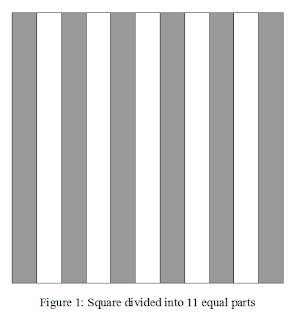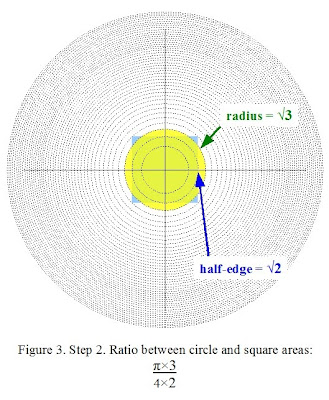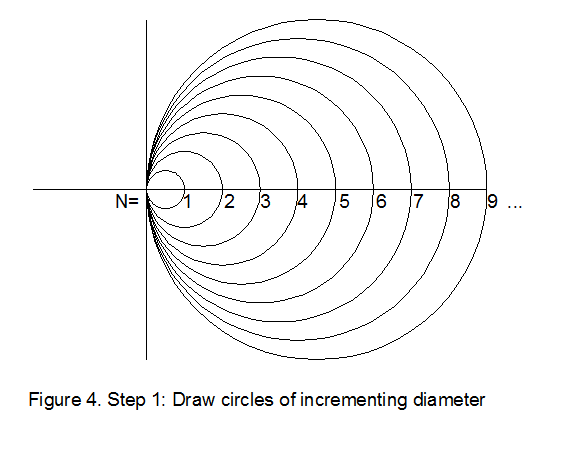## Sunday, March 28, 2010

### Wallis product for nested equilateral trianglesI'm discovering the Wallis product thanks to an approach involving geometry of areas. In order to relate that to what has already been written on the subject, here are some works which I would like to study in the coming time:
Maybe you have other suggestions on this subject.

Meanwhile, I tried to draw equilateral triangles in the exhaustion scheme of nested circles. We start from the concentric rings of same area as described in Figure 4 of the Variations of circular area division into equal parts post. Remember the inner circle has radius 1 and area π. Each subsequent Nth circle has radius square root of N (noted √N) and area Nπ. Then we can draw an equilateral triangle whose sides are tangent to the first circle. The neat thing about this procedure is that we can read out the pertaining numerical values right on the figure. Figure 1 shows us that the area of the equilateral triangle is 3√3. So the ratio between the outscribed equilateral triangle area and the circle area is equal to 3√3/π.

By drawing equilateral triangles tangent to circles of incrementing area Nπ, we discover the nested equal area division for equilateral triangles: the area between each equilateral triangle is equal to 3√3 (see Figure 2, you can click on the figure to view it enlarged). Among other interesting features, this figure contains the table of 4. For each triangle outscribing a circle of radius √N, its vertices are located on the circle of order 4×N.

If we outscribe alternately a circle, a triangle, a circle, a triangle, a circle, etc., the circles (and the vertices of the triangles) go through the subsequent powers of 4, see Figure 3. By changing the form of the triangle, we have access to other tables and powers. It seems there are plenty of properties that are hidden in this structure.
Now, we can try the Wallis exhaustion scheme on the nested circle and triangle structure. The procedure apparently goes something like:
Step 1. Draw the initial circle and triangle with area ratio 3√3/4π.
Step 2. A better fit would be to inscribe both figures in higher orders of the nested structure. But the area of the triangle is too small with respect to the circle. So we must enlarge the area of the triangle more than the circle. We enlarge the area of the triangle by 3 while leaving unchanged the area of the circle. This is illustrated in the figure below.
Step 3. But now the area of the triangle is too large with respect to the circular area. So for this fit, we must enlarge the area of the triangle a little less than the area of the circle. We therefore enlarge the area of the triangle by 3, while enlarging the area of the circle by 4, as illustrated below.
Step 4. But now the area of the triangle is too small with respect to the circular area. So for the next fit, we must enlarge the area of the triangle a little more than the area of the circle. We therefore enlarge the area of the circle by 6, while enlarging the area of the circle by 5, as illustrated below.
This exhaustion scheme that makes the area of equilateral triangles alternately larger, smaller, larger, smaller, etc... than the circular area can be written as:
This formula corresponds to an infinite product approximating π, which I've found in a French book Le fascinant nombre π by Jean-Paul Delahaye but I couldn't find it mentioned elsewhere. As approximation method, it is about twice as fast as Wallis' original product. This equilateral triangle exhaustion product is probably a special case in a large family of Wallis products, each of which pertaining to a particular geometrical configuration from which one tries to approximate π. The starting and recurrence conditions depend on the form and the initial area ratio between the polygon and the circle. The rules governing this exhaustion scheme in the general case still elude me.

## Sunday, March 21, 2010

### Exhaustion of nested squares and Wallis productWhen one is asked to divide a square into N equal area parts, we commonly think of dividing a square into N equal rectangles. Figure 1 shows an example for N=11.

There is another general solution to that problem, in particular we could wrap it up in an enigma: is it possible to divide a square into N square-shaped parts of equal area, N being any integer?

The nested circles structure described in Figure 4 of the Variations of circular area division into equal parts post suggests the following solution.

Step 1. Starting from a circle of radius 1, area π, draw circles of incrementing area 2π, 3π, 4π, 5π, 6π, and so on... The resulting concentric rings have all the same area π.Step 2. For each circle, circumscribe a square. The resulting squares have area 4, 8, 12, 16, 20, 24, and so on...Step 3. Conclusion: the areas between each nested squares are equal. The figure illustrates the case for N=11.So here we have a compass and ruler solution for dividing a square into N equal area parts. An interesting corollary is that this division into N equal parts can be applied to any 2D-figure, whether it be a triangle, a pentagon, any polygon, or even arbitrary drawings, provided that it be circumscribed (or inscribed) in a circle. As an answer to a comment on the preceding post, why not divide Leonardo da Vinci's Vitruvian man into N nested Vitruvian men? There are certainly other applications, one of them has been suggested to me by another comment on the preceding post.

In order to calculate an approximate value of π, Archimedes used a method of exhaustion which consists in inscribing a circle between two identically shaped polygons. Increasing gradually the number of sides of the polygon, the shape of the polygon approaches the shape of a circle. This geometrical approach is at the origin of his numerical approximation of π. Many other ways have been developed to approximate π, especially using infinite series. Geometrical representations of these series are rarely available, probably because no direct link has been found between them and the circle. The benefit of the nested circle and square structure of Figure 2 is that it suggests alternative methods of exhaustion.

For example, we could try to equate the area of a circle to the area of a square in this nested structure. This yields an approximation for squaring the circle (or circling the square). For a circle of radius 1, the area of the circle is π, the area of the circumscribed square is 4. The ratio between both areas is therefore π/4. The procedure to let that ratio go to 1 then follows the following steps.

Step 1. Draw the initial circle and square with ratio π/4.
Step 2. A better fit would be to inscribe both figures in higher orders of the nested structure. But the area of the circle is too small with respect to the square. So, we must enlarge the area of the circle a little more than the square. We therefore enlarge the area of the circle by 3 while enlarging the area of the square by 2, which is illustrated in the figure below.Step 3. But now the area of the circle is too large with respect to the square. So for this fit, we must enlarge the area of the circle a little less than the area of the square. We therefore enlarge the area of the circle by 3, while enlarging the area of the square by 4, as illustrated below.Step 4. But now the area of the circle is again too small with respect to the square. So for the next fit, we must enlarge the area of the circle a little more than the area of the square. We therefore enlarge the area of the circle by 5, while enlarging the area of the square by 4, as illustrated below.
Step 5. As after step 2, the area of the circle is too large with respect to the square... and I become a bit exhausted by drawing at exponentially growing scales;-) but I hope I've shown enough to suggest the continuation of this exhaustion scheme. You can of course rescale the figures, keeping track of the successive numbers and their roots on the figures.

The described method of exhaustion searches for a limit by alternately letting the circular area become larger, smaller, larger, smaller, etc... than the square area, but each time with a reduced fraction. As a limit it can be expressed by the following equation:It seems we have gone through Wallis' product, which is usually disconnected from any geometrical approach.
______________________________________________________

Profound study of nature is the most fertile source of mathematical discoveries. ~ Joseph Fourier

## Sunday, March 14, 2010

### Variations on dividing circular area into equal partsToday is π-day. Investigating the properties of a circle is one of my favorite hobbies, so this is an appropriate event for some discussion on the circle. I love drawing circles and lines on a piece of paper. It's fascinating to discover natural laws in the circle. At each of my drawings, I gain some new insghts. Recently I came across a Cut The Knot post presenting an inventive way to divide a circular area into any number of equal parts, with compass and ruler. I googled a little more over it and curiously, it seems that the presented yin and yang-like solution is the only one that has been presented (if you know of other published references, I would be eager to hear about). It appeared in a Mathematical Association book in 1995 but I didn't find any other reference to solutions of this problem. This is surprising, because area division is a fundamental problem with broad applications in this world where sharing physical resources equally is a necessary guarantee for peace. Playing a bit with the elementary components of the yin and yang pattern gives related solutions, of which a random one is sketched in Figure 1.I convinced myself that the most general solution is in fact a quite natural and intuitive one, because it figures the way how circular patterns often grow in nature, like the annual rings in wood.Other patterns also give some insight in various ways to divide a circular area into parts, like the beautiful quintuple emulsions formed with microfluidics of the figured image (courtesy of A. Abate/ Harvard University).

The general rule to have a circular area divided equally into N of such nested circles is that, for a starting circle of radius 1, the surrounding Nth circle radius is the square root of N (noted √N).

The first circle has radius 1, area π.
The second circle has radius √2, area 2π.
The third circle has radius √3, area 3π.
The fourth circle has radius √4 (= 2), area 4π.
And so on...

Square roots can be easily drawn with compass and ruler, so the same for nested circles with radius equal to √N, where N is incremented from 1 to any positive integer value. Figure 4 shows a convenient procedure for N=1 to 9:

Step 1. Draw nested circles with diameter 1 to 9 whose left diameter endpoints coincide.
Step 2. Draw a perpendicular to the diameter direction at the intersection of the right endpoint of the circle with diameter 1.
Step 3. Draw circles of radius equal to √N thanks to intersections of first circles with perpendicular at N=1.
Step 4. Erase lines and circles of intermediate steps. The obtained outer circle is divided into N equal parts each of area π.Now, from this pattern, it is possible to start a set of variations on circular area division, because the nested circles are whole, contrarily to the yin and yang pattern. We may for example start to divide the circle of area 2π into two equal half-circles and shift those components randomly (see Figure 5). Or we may start to divide the circle of area 3π into equal parts, etc. I'm sure anyone can find original new ways to arrange and transform all those equal parts, in order to gain some new intuitions on the circle.Happy π-day!